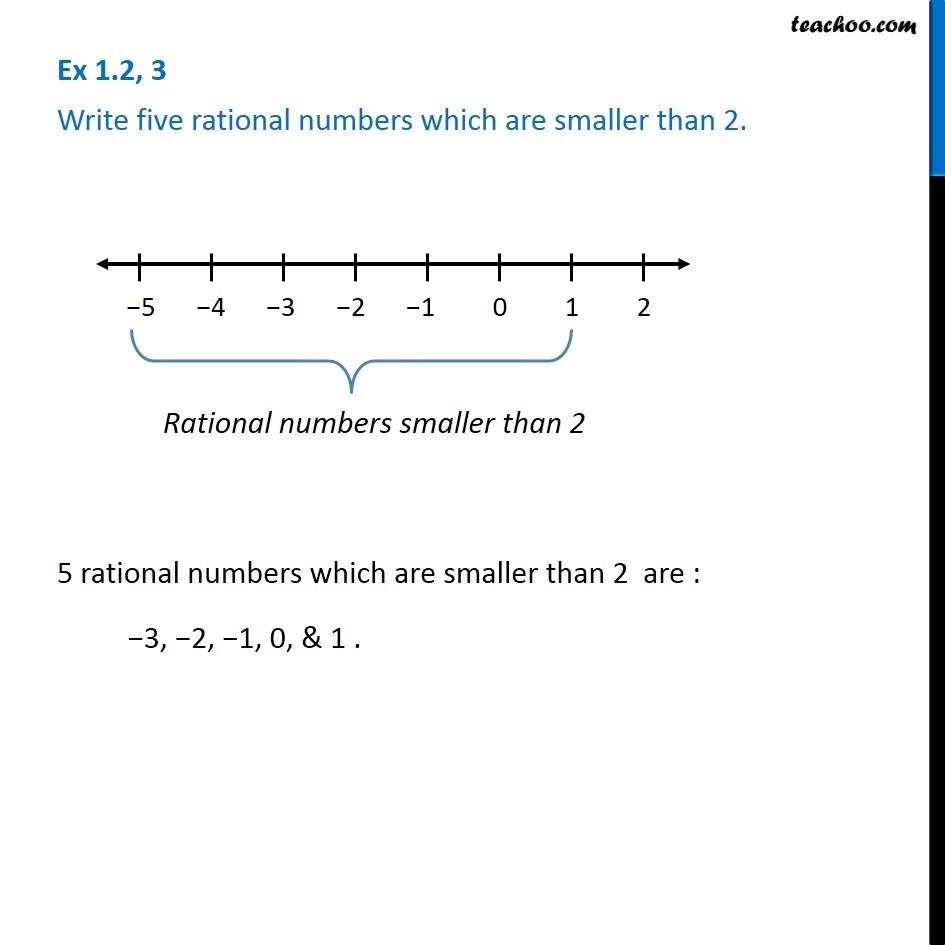1. Chapter 1 Class 8 Rational Numbers
2. Serial order wise
3. Ex 1.2

Transcript

Ex 1.2, 3 - Chapter 1 Class 8 Rational Numbers - NCERT Solutions Write 5 rational numbers smaller than 2? To find rational numbers, let us draw a number line Now, numbers on the left of number line are smaller than 2 Thus, 5 rational numbers which are smaller than 2 are : 3, 2, 1, 0, & 1 .

Ex 1.2

Chapter 1 Class 8 Rational Numbers
Serial order wise